• Python 使用Opencv实现图像特征检测与匹配

万次阅读 多人点赞 2018-06-13 11:36:58
本人新书《玩转Python网络爬虫》，可在天猫、京东等商城搜索查阅，项目深入浅出，适合爬虫初学者或者是已经有一些网络爬虫编写经验，但希望更加全面、深入理解Python爬虫的开发人员。...角检测...

----------欢迎加入学习交流QQ群：657341423

特征检测是计算机对一张图像中最为明显的特征进行识别检测并将其勾画出来。大多数特征检测都会涉及图像的角点、边和斑点的识别、或者是物体的对称轴。
角点检测 是由Opencv的cornerHarris函数实现，其他函数参数说明如下：

cv2.cornerHarris(src=gray, blockSize=9, ksize=23, k=0.04)
# cornerHarris参数：
# src - 数据类型为 float32 的输入图像。
# blockSize - 角点检测中要考虑的领域大小。
# ksize - Sobel 求导中使用的窗口大小
# k - Harris 角点检测方程中的自由参数,取值参数为 [0,04,0.06].

以国际象棋为例，这是计算机视觉最为常见的分析对象，如图所示：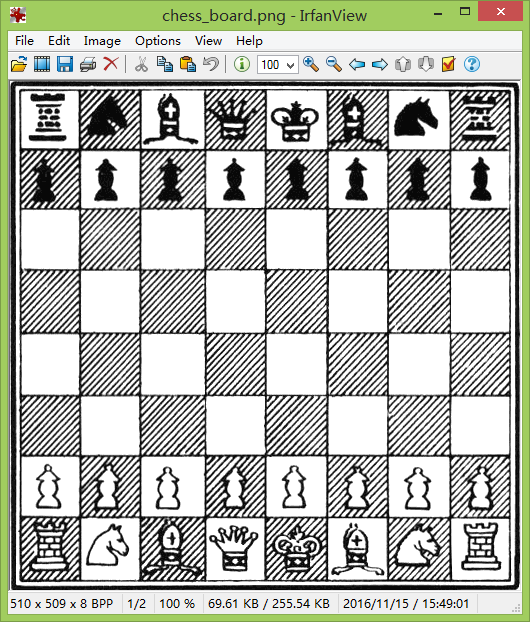角点检测代码如下：

import cv2
import numpy as np

gray = cv2.cvtColor(img, cv2.COLOR_BGR2GRAY)
# cornerHarris函数图像格式为 float32 ，因此需要将图像转换 float32 类型
gray = np.float32(gray)
# cornerHarris参数：
# src - 数据类型为 float32 的输入图像。
# blockSize - 角点检测中要考虑的领域大小。
# ksize - Sobel 求导中使用的窗口大小
# k - Harris 角点检测方程中的自由参数,取值参数为 [0,04,0.06].
dst = cv2.cornerHarris(src=gray, blockSize=9, ksize=23, k=0.04)
# 变量a的阈值为0.01 * dst.max()，如果dst的图像值大于阈值，那么该图像的像素点设为True，否则为False
# 将图片每个像素点根据变量a的True和False进行赋值处理，赋值处理是将图像角点勾画出来
a = dst>0.01 * dst.max()
img[a] = [0, 0, 255]
# 显示图像
while (True):
cv2.imshow('corners', img)
if cv2.waitKey(120) & 0xff == ord("q"):
break
cv2.destroyAllWindows()

运行代码，结果如图所示：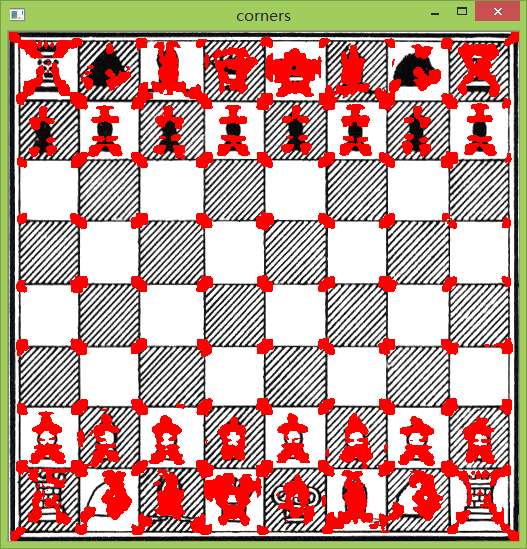但有时候，图像的像素大小对角点存在一定的影响。比如图像越小，角点看上去趋向近似一条直线，这样很容易造成角点的丢失。如果按照上述的检测方法，会造成角点检测结果不相符，因此引入DoG和SIFT算法进行检测Opencv的SIFT类是DoG和SIFT算法组合。
DoG是对同一图像使用不同高斯滤波器所得的结果。
SIFT是通过一个特征向量来描述关键点周围区域的情况。
我们以下图为例：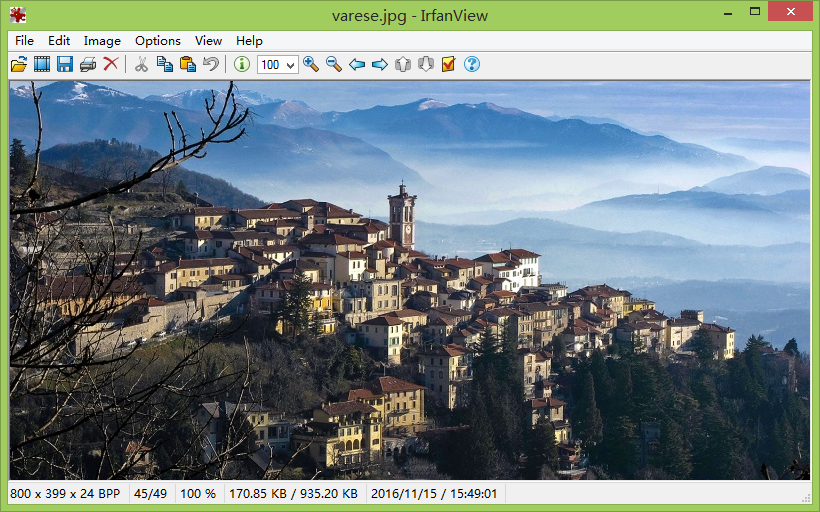import cv2
# 读取图片并灰度处理
imgpath = 'varese.jpg'
gray = cv2.cvtColor(img, cv2.COLOR_BGR2GRAY)
# 创建SIFT对象
sift = cv2.xfeatures2d.SIFT_create()
# 将图片进行SURF计算，并找出角点keypoints，keypoints是检测关键点
# descriptor是描述符，这是图像一种表示方式，可以比较两个图像的关键点描述符，可作为特征匹配的一种方法。
keypoints, descriptor = sift.detectAndCompute(gray, None)

# cv2.drawKeypoints() 函数主要包含五个参数：
# image: 原始图片
# keypoints：从原图中获得的关键点，这也是画图时所用到的数据
# outputimage：输出
# color：颜色设置，通过修改（b,g,r）的值,更改画笔的颜色，b=蓝色，g=绿色，r=红色。
# flags：绘图功能的标识设置，标识如下：
# cv2.DRAW_MATCHES_FLAGS_DEFAULT  默认值
# cv2.DRAW_MATCHES_FLAGS_DRAW_RICH_KEYPOINTS
# cv2.DRAW_MATCHES_FLAGS_DRAW_OVER_OUTIMG
# cv2.DRAW_MATCHES_FLAGS_NOT_DRAW_SINGLE_POINTS
img = cv2.drawKeypoints(image=img, outImage=img, keypoints = keypoints, flags=cv2.DRAW_MATCHES_FLAGS_DEFAULT, color = (51, 163, 236))

# 显示图片
cv2.imshow('sift_keypoints', img)
while (True):
if cv2.waitKey(120) & 0xff == ord("q"):
break
cv2.destroyAllWindows()

运行代码，结果如图所示：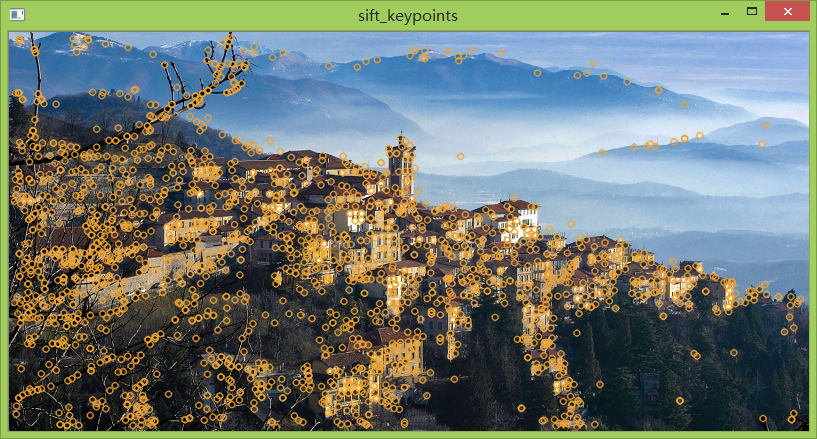除了SIFT算法检测之外，还有SURF特征检测算法，比SIFT算法快，并吸收了SIFT算法的思想。SURF采用Hessian算法检测关键点，而SURF是提取特征，这个与SIFT很像。Opencv的SURF类是Hessian算法和SURF算法组合。我们根据SIFT的代码进行修改，代码如下：

import cv2
# 读取图片并灰度处理
imgpath = 'varese.jpg'
gray = cv2.cvtColor(img, cv2.COLOR_BGR2GRAY)
# 创建SURF对象，对象参数float(4000)为阈值，阈值越高，识别的特征越小。
sift = cv2.xfeatures2d.SURF_create(float(4000))
# 将图片进行SURF计算，并找出角点keypoints，keypoints是检测关键点
# descriptor是描述符，这是图像一种表示方式，可以比较两个图像的关键点描述符，可作为特征匹配的一种方法。
keypoints, descriptor = sift.detectAndCompute(gray, None)

# cv2.drawKeypoints() 函数主要包含五个参数：
# image: 原始图片
# keypoints：从原图中获得的关键点，这也是画图时所用到的数据
# outputimage：输出
# color：颜色设置，通过修改（b,g,r）的值,更改画笔的颜色，b=蓝色，g=绿色，r=红色。
# flags：绘图功能的标识设置，标识如下：
# cv2.DRAW_MATCHES_FLAGS_DEFAULT  默认值
# cv2.DRAW_MATCHES_FLAGS_DRAW_RICH_KEYPOINTS
# cv2.DRAW_MATCHES_FLAGS_DRAW_OVER_OUTIMG
# cv2.DRAW_MATCHES_FLAGS_NOT_DRAW_SINGLE_POINTS
img = cv2.drawKeypoints(image=img, outImage=img, keypoints = keypoints, flags=cv2.DRAW_MATCHES_FLAGS_DEFAULT, color = (51, 163, 236))

# 显示图片
cv2.imshow('sift_keypoints', img)
while (True):
if cv2.waitKey(120) & 0xff == ord("q"):
break
cv2.destroyAllWindows()

上述代码我们只修改sift = cv2.xfeatures2d.SURF_create(float(4000))即可实现SURF特征检测算法。运行结果如图所示：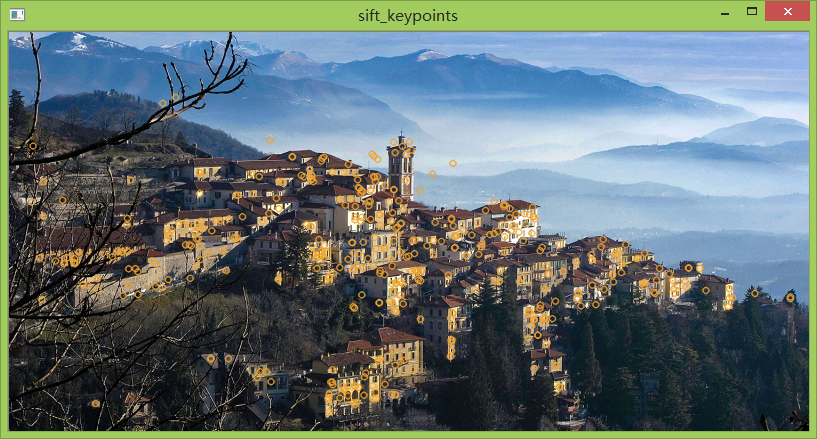对比SURF和SIFT算法，ORB算法更处于起步阶段，在2011年才首次发布。但比前两者的速度更快。ORB基于FAST关键点检测和BRIEF的描述符技术相结合，因此我们先了解FAST和BRIEF。
FAST：特征检测算法。
BRIEF：只是一个描述符，这是图像一种表示方式，可以比较两个图像的关键点描述符，可作为特征匹配的一种方法。
暴力匹配：比较两个描述符并产生匹配结果。
在上述的例子中，我们只是将检测的关键点进行勾画，在这例子中，将使用ORB检测关键点之外，还将两图进行匹配，匹配的图像如下：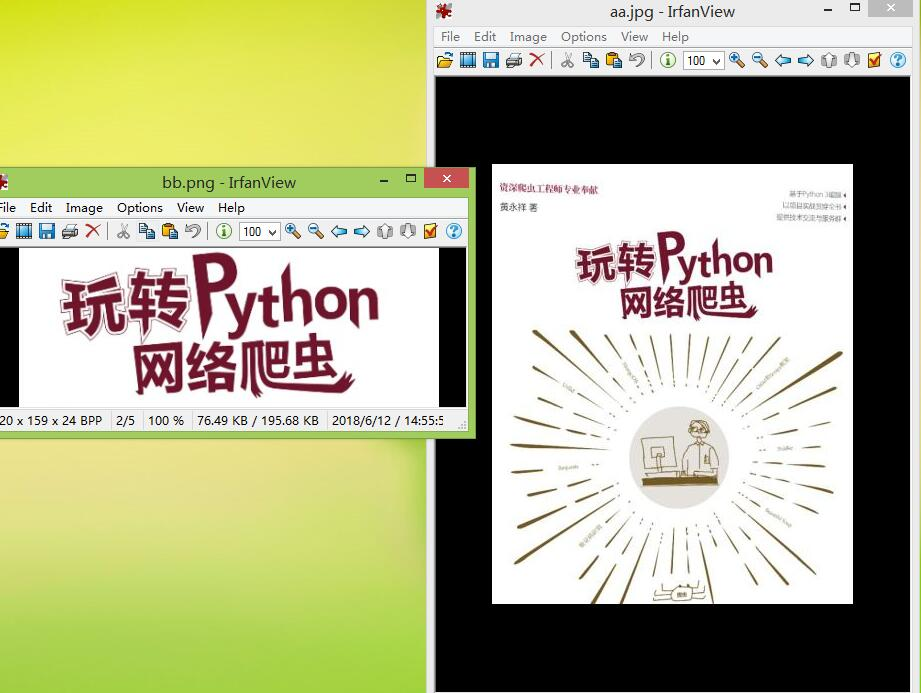实现方法：首先分别对两图进行ORB处理，然后将两图的关键点进行暴力匹配。具体代码如下：
# ORB算法实现特征检测+暴力匹配

import numpy as np
import cv2
from matplotlib import pyplot as plt

# 读取图片内容

# 使用ORB特征检测器和描述符，计算关键点和描述符
orb = cv2.ORB_create()
kp1, des1 = orb.detectAndCompute(img1,None)
kp2, des2 = orb.detectAndCompute(img2,None)

# 暴力匹配BFMatcher，遍历描述符，确定描述符是否匹配，然后计算匹配距离并排序
# BFMatcher函数参数：
# normType：NORM_L1, NORM_L2, NORM_HAMMING, NORM_HAMMING2。
# NORM_L1和NORM_L2是SIFT和SURF描述符的优先选择，NORM_HAMMING和NORM_HAMMING2是用于ORB算法
bf = cv2.BFMatcher(normType=cv2.NORM_HAMMING, crossCheck=True)
matches = bf.match(des1,des2)
matches = sorted(matches, key = lambda x:x.distance)
# matches是DMatch对象，具有以下属性：
# DMatch.distance - 描述符之间的距离。 越低越好。
# DMatch.trainIdx - 训练描述符中描述符的索引
# DMatch.queryIdx - 查询描述符中描述符的索引
# DMatch.imgIdx - 训练图像的索引。

# 使用plt将两个图像的匹配结果显示出来
img3 = cv2.drawMatches(img1=img1,keypoints1=kp1,img2=img2,keypoints2=kp2, matches1to2=matches, outImg=img2, flags=2)
plt.imshow(img3),plt.show()

运行结果如图所示：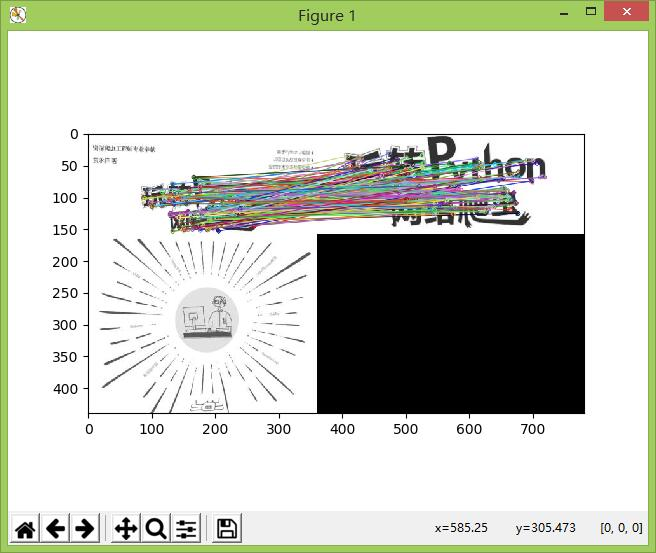# SURF和SIFT算法+暴力匹配
暴力匹配BFMatcher是一种匹配方法，只要提供两个关键点即可实现匹配。若将上述例子改为SURF和SIFT算法，只需修改以下代码：

将orb = cv2.ORB_create()改为
orb = cv2.xfeatures2d.SURF_create(float(4000))

将bf = cv2.BFMatcher(normType=cv2.NORM_HAMMING, crossCheck=True)改为
bf = cv2.BFMatcher(normType=cv2.NORM_L1, crossCheck=True)

# 获取匹配关键点的坐标位置
在上述例子中，matches是DMatch对象，DMatch是以列表的形式表示，每个元素代表两图能匹配得上的点。如果想获取某个点的坐标位置，在上述例子添加以下代码：

# 由于匹配顺序是：matches = bf.match(des1,des2)，先des1后des2。
# 因此，kp1的索引由DMatch对象属性为queryIdx决定，kp2的索引由DMatch对象属性为trainIdx决定

# 获取aa.jpg的关键点位置
x,y = kp1[matches.queryIdx].pt
cv2.rectangle(img1, (int(x),int(y)), (int(x) + 5, int(y) + 5), (0, 255, 0), 2)
cv2.imshow('a', img1)

# 获取bb.png的关键点位置
x,y = kp2[matches.trainIdx].pt
cv2.rectangle(img2, (int(x1),int(y1)), (int(x1) + 5, int(y1) + 5), (0, 255, 0), 2)
cv2.imshow('b', img2)

# 使用plt将两个图像的第一个匹配结果显示出来
img3 = cv2.drawMatches(img1=img1,keypoints1=kp1,img2=img2,keypoints2=kp2, matches1to2=matches[:1], outImg=img2, flags=2)
plt.imshow(img3),plt.show()

运行结果如图所示：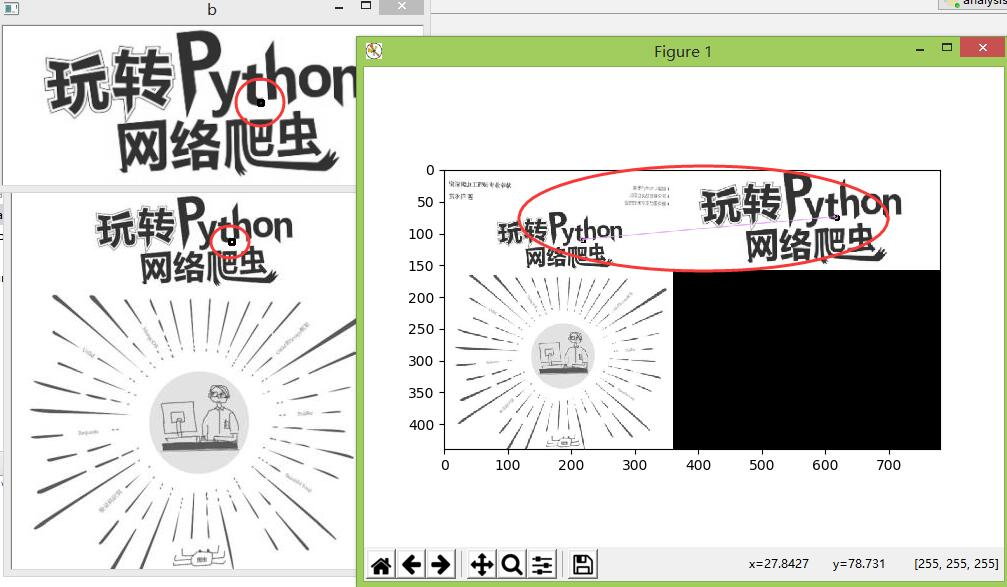上述讲到的暴力匹配是使用BFMatcher匹配器实现的，然后由match函数实现匹配。接下来讲解K-最近邻匹配（KNN），并在BFMatcher匹配下实现。在所有机器学习的算法中，KNN可能是最为简单的算法。针对上述例子，改为KNN匹配，实现代码如下：

import numpy as np
import cv2
from matplotlib import pyplot as plt

# 读取图片内容

# 使用ORB特征检测器和描述符，计算关键点和描述符
orb = cv2.ORB_create()
kp1, des1 = orb.detectAndCompute(img1,None)
kp2, des2 = orb.detectAndCompute(img2,None)

# 暴力匹配BFMatcher，遍历描述符，确定描述符是否匹配，然后计算匹配距离并排序
# BFMatcher函数参数：
# normType：NORM_L1, NORM_L2, NORM_HAMMING, NORM_HAMMING2。
# NORM_L1和NORM_L2是SIFT和SURF描述符的优先选择，NORM_HAMMING和NORM_HAMMING2是用于ORB算法
bf = cv2.BFMatcher(normType=cv2.NORM_HAMMING, crossCheck=True)
# knnMatch 函数参数k是返回符合匹配的个数，暴力匹配match只返回最佳匹配结果。
matches = bf.knnMatch(des1,des2,k=1)

# 使用plt将两个图像的第一个匹配结果显示出来
# 若使用knnMatch进行匹配，则需要使用drawMatchesKnn函数将结果显示
img3 = cv2.drawMatchesKnn(img1=img1,keypoints1=kp1,img2=img2,keypoints2=kp2, matches1to2=matches, outImg=img2, flags=2)
plt.imshow(img3),plt.show()

最后是介绍FLANN匹配，相对暴力匹配BFMatcher来讲，这匹配算法比较准确、快速和使用方便。FLANN具有一种内部机制，可以根据数据本身选择最合适的算法来处理数据集。值得注意的是，FLANN匹配器只能使用SURF和SIFT算法。
FLANN实现方式如下：

import numpy as np
import cv2
from matplotlib import pyplot as plt

# 只使用SIFT 或 SURF 检测角点
sift = cv2.xfeatures2d.SIFT_create()
# sift = cv2.xfeatures2d.SURF_create(float(4000))
kp1, des1 = sift.detectAndCompute(queryImage,None)
kp2, des2 = sift.detectAndCompute(trainingImage,None)

# 设置FLANN匹配器参数
# algorithm设置可参考https://docs.opencv.org/3.1.0/dc/d8c/namespacecvflann.html
indexParams = dict(algorithm=0, trees=5)
searchParams = dict(checks=50)
# 定义FLANN匹配器
flann = cv2.FlannBasedMatcher(indexParams,searchParams)
# 使用 KNN 算法实现匹配
matches = flann.knnMatch(des1,des2,k=2)

matchesMask = [[0,0] for i in range(len(matches))]

# 去除错误匹配
for i,(m,n) in enumerate(matches):
if m.distance < 0.7*n.distance:

# 将图像显示
# singlePointColor是勾画关键点
drawParams = dict(matchColor = (0,255,0),
singlePointColor = (255,0,0),
flags = 0)
resultImage = cv2.drawMatchesKnn(queryImage,kp1,trainingImage,kp2,matches,None,**drawParams)
plt.imshow(resultImage,),plt.show()

运行结果如图所示：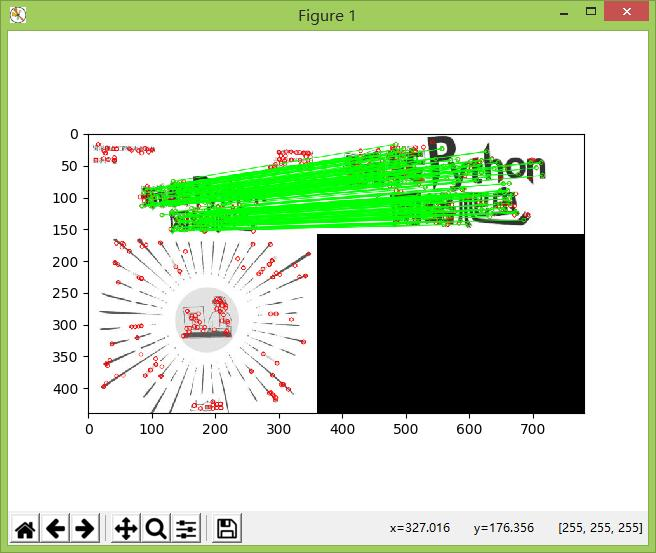FLANN的单应性匹配，单应性是一个条件，该条件表面当两幅图像中的一副出像投影畸变时，他们还能匹配。FLANN的单应性实现代码如下：

import numpy as np
import cv2
from matplotlib import pyplot as plt

MIN_MATCH_COUNT = 10

# 使用SIFT检测角点
sift = cv2.xfeatures2d.SIFT_create()
# 获取关键点和描述符
kp1, des1 = sift.detectAndCompute(img1,None)
kp2, des2 = sift.detectAndCompute(img2,None)

# 定义FLANN匹配器
index_params = dict(algorithm = 1, trees = 5)
search_params = dict(checks = 50)
flann = cv2.FlannBasedMatcher(index_params, search_params)
# 使用KNN算法匹配
matches = flann.knnMatch(des1,des2,k=2)

# 去除错误匹配
good = []
for m,n in matches:
if m.distance < 0.7*n.distance:
good.append(m)

# 单应性
if len(good)>MIN_MATCH_COUNT:
# 改变数组的表现形式，不改变数据内容，数据内容是每个关键点的坐标位置
src_pts = np.float32([ kp1[m.queryIdx].pt for m in good ]).reshape(-1,1,2)
dst_pts = np.float32([ kp2[m.trainIdx].pt for m in good ]).reshape(-1,1,2)
# findHomography 函数是计算变换矩阵
# 参数cv2.RANSAC是使用RANSAC算法寻找一个最佳单应性矩阵H，即返回值M
M, mask = cv2.findHomography(src_pts, dst_pts, cv2.RANSAC,5.0)
# ravel方法将数据降维处理，最后并转换成列表格式
# 获取img1的图像尺寸
h,w = img1.shape
# pts是图像img1的四个顶点
pts = np.float32([[0,0],[0,h-1],[w-1,h-1],[w-1,0]]).reshape(-1,1,2)
# 计算变换后的四个顶点坐标位置
dst = cv2.perspectiveTransform(pts,M)

# 根据四个顶点坐标位置在img2图像画出变换后的边框
img2 = cv2.polylines(img2,[np.int32(dst)],True,(255,0,0),3, cv2.LINE_AA)

else:
print("Not enough matches are found - %d/%d") % (len(good),MIN_MATCH_COUNT)

# 显示匹配结果
draw_params = dict(matchColor = (0,255,0), # draw matches in green color
singlePointColor = None,
flags = 2)
img3 = cv2.drawMatches(img1,kp1,img2,kp2,good,None,**draw_params)
plt.imshow(img3, 'gray'),plt.show()

运行结果如下所示：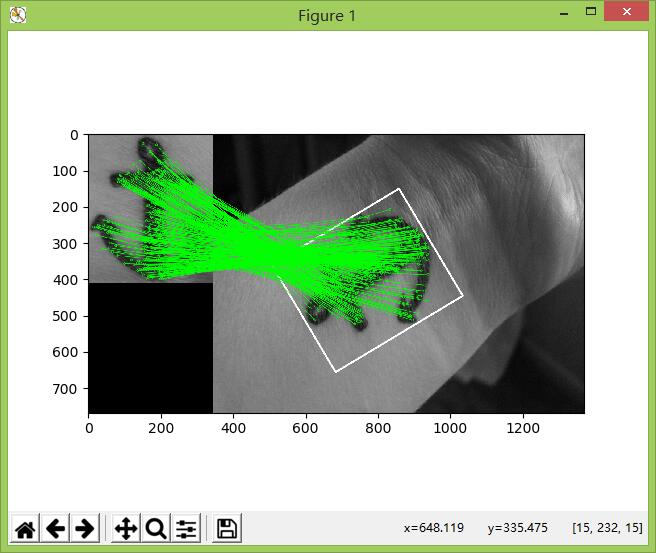单应性实际应用
从上述的例子可以看到，单应性是在两图片匹配的时候，其中某一图片发生变换处理，变换后图像会呈现一种立体空间的视觉效果，图像发生变换程度称为变换矩阵。以下例子将图像中的书本替换成其他书本，例子中所使用图片如下：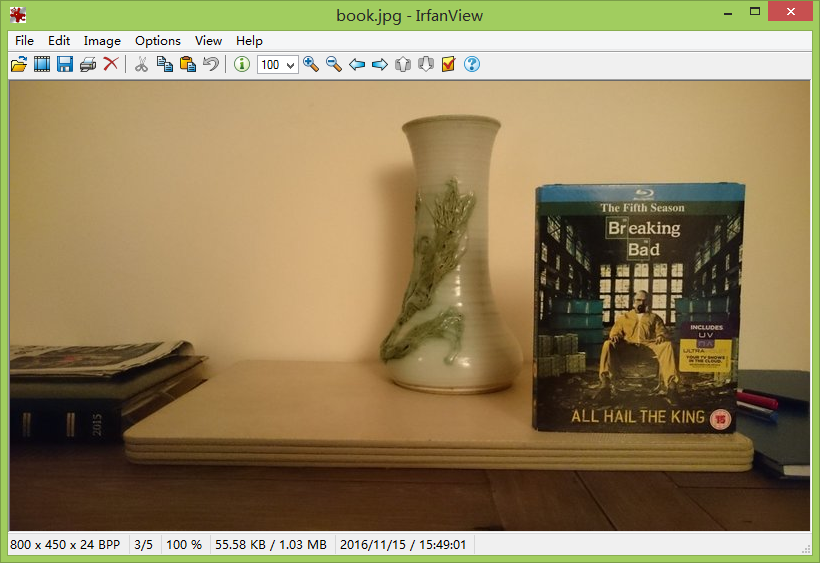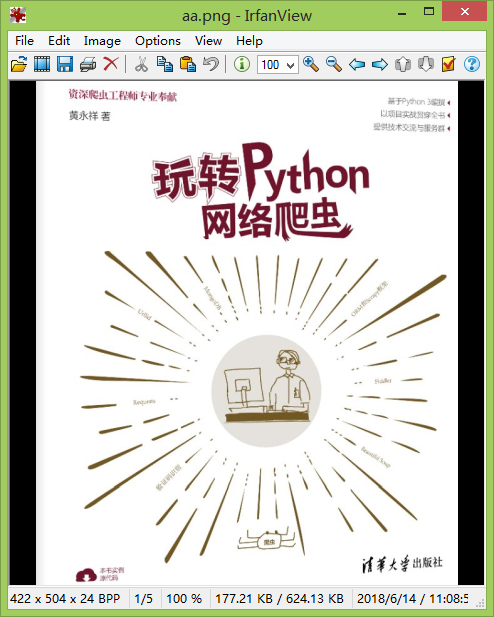我们根据图1和图2计算变换矩阵，然后通过变换矩阵将图3进行变换，最后将图3加入到图1中，实现图片替换。实现代码如下：

import numpy as np
import cv2
from matplotlib import pyplot as plt

# 使用SIFT检测角点
sift = cv2.xfeatures2d.SIFT_create()
# 获取关键点和描述符
kp1, des1 = sift.detectAndCompute(img1,None)
kp2, des2 = sift.detectAndCompute(img2,None)

# 定义FLANN匹配器
index_params = dict(algorithm = 1, trees = 5)
search_params = dict(checks = 50)
flann = cv2.FlannBasedMatcher(index_params, search_params)
# 使用KNN算法匹配
matches = flann.knnMatch(des1,des2,k=2)

# 去除错误匹配
good = []
for m,n in matches:
if m.distance < 0.7*n.distance:
good.append(m)

# 单应性实际应用
# 改变数组的表现形式，不改变数据内容，数据内容是每个关键点的坐标位置
src_pts = np.float32([ kp1[m.queryIdx].pt for m in good ]).reshape(-1,1,2)
dst_pts = np.float32([ kp2[m.trainIdx].pt for m in good ]).reshape(-1,1,2)
# findHomography 函数是计算变换矩阵
# 参数cv2.RANSAC是使用RANSAC算法寻找一个最佳单应性矩阵H，即返回值M
M, mask = cv2.findHomography(src_pts, dst_pts, cv2.RANSAC,5.0)
# 获取img1的图像尺寸
h,w = img1.shape
# pts是图像img1的四个顶点
pts = np.float32([[0,0],[0,h-1],[w-1,h-1],[w-1,0]]).reshape(-1,1,2)
# 计算变换后的四个顶点坐标位置
dst = cv2.perspectiveTransform(pts,M)

# 图片替换
# 降维处理
b = np.int32(dst).reshape(4, 2)
x,y = img2.shape
# 根据变换矩阵将图像img3进行变换处理
res = cv2.warpPerspective(img3, M, (y,x))
img_temp = img2.copy()
# 将图像img2的替换区域进行填充处理
cv2.fillConvexPoly(img_temp, b, 0)
# 将变换后的img3图像替换到图像img2
cv2.imshow('bb',img_temp)
res = img_temp + res
cv2.imshow('aa',res)
plt.imshow(res),plt.show()

运行结果如图所示：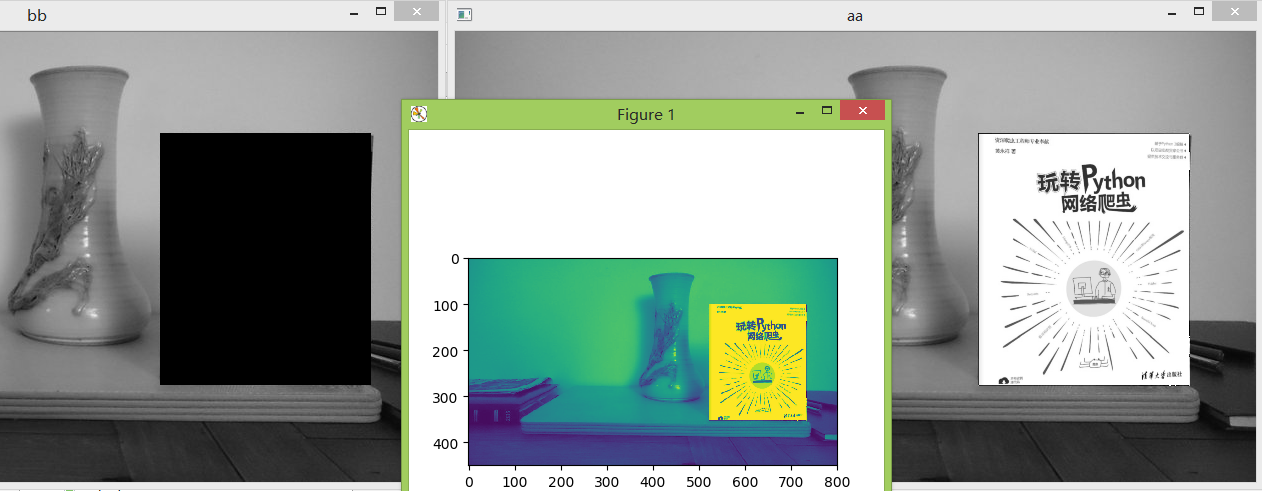从结果可以看到，替换后的图像周边出现黑色线条，这是正常的现象。在上图最左边的图bb可以看到，黑色区域是由图1和图2检测匹配所得的结果，如果匹配结果会存在一定的误差，这个误差是由多个因素所导致的。

在实际中，我们根据一张图片在众多的图片中查找匹配率最高的图片。如果按照上面的例子，也可以实现，但每次匹配时都需要重新检测图片的特征数据，这样会导致程序运行效率。因此，我们可以将图片的特征数据进行保存，每次匹配时，只需读取特征数据进行匹配即可。我们以下图为例：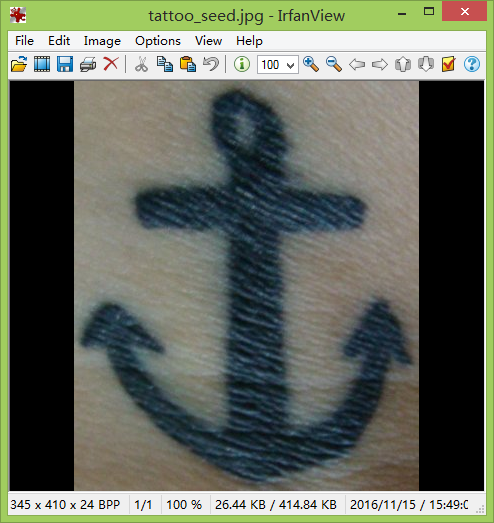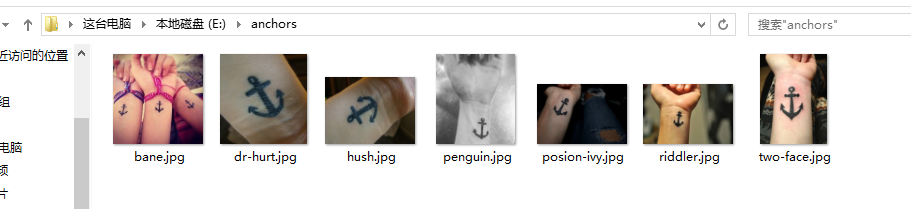我们根据图1在图2中查找最佳匹配的图片。首先获取图2的全部图片的特征数据，将代码保存在features.py

import cv2
import numpy as np
from os import walk
from os.path import join

def create_descriptors(folder):
files = []
for (dirpath, dirnames, filenames) in walk(folder):
files.extend(filenames)
for f in files:
if '.jpg' in f:
save_descriptor(folder, f, cv2.xfeatures2d.SIFT_create())

def save_descriptor(folder, image_path, feature_detector):
# 判断图片是否为npy格式
if image_path.endswith("npy"):
return
# 读取图片并检查特征
keypoints, descriptors = feature_detector.detectAndCompute(img, None)
# 设置文件名并将特征数据保存到npy文件
descriptor_file = image_path.replace("jpg", "npy")
np.save(join(folder, descriptor_file), descriptors)

if __name__=='__main__':
path = 'E:\\anchors'
create_descriptors(path)

运行代码，结果如图所示：我们将图片的特征数据保存在npy文件。下一步是根据图1与这些特征数据文件进行匹配，从而找出最佳匹配的图片。代码存在matching.py

from os.path import join
from os import walk
import numpy as np
import cv2

folder = 'E:\\anchors'
descriptors = []
# 获取特征数据文件名
for (dirpath, dirnames, filenames) in walk(folder):
for f in filenames:
if f.endswith("npy"):
descriptors.append(f)
print(descriptors)

# 使用SIFT算法检查图像的关键点和描述符
sift = cv2.xfeatures2d.SIFT_create()
query_kp, query_ds = sift.detectAndCompute(query, None)

# 创建FLANN匹配器
index_params = dict(algorithm=0, trees=5)
search_params = dict(checks=50)
flann = cv2.FlannBasedMatcher(index_params, search_params)

potential_culprits = {}
for d in descriptors:
# 将图像query与特征数据文件的数据进行匹配
matches = flann.knnMatch(query_ds, np.load(join(folder, d)), k=2)
# 清除错误匹配
good = []
for m, n in matches:
if m.distance < 0.7 * n.distance:
good.append(m)
# 输出每张图片与目标图片的匹配数目
print("img is %s ! matching rate is (%d)" % (d, len(good)))
potential_culprits[d] = len(good)

# 获取最多匹配数目的图片
max_matches = None
potential_suspect = None
for culprit, matches in potential_culprits.items():
if max_matches == None or matches > max_matches:
max_matches = matches
potential_suspect = culprit

print("potential suspect is %s" % potential_suspect.replace("npy", "").upper())

代码运行后，输出结果如图所示：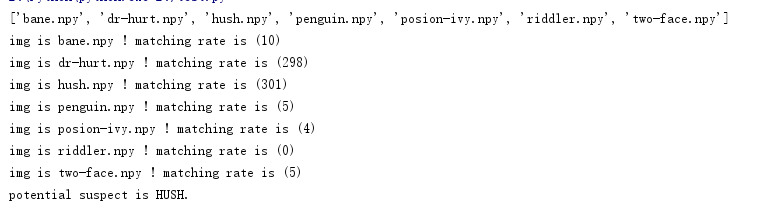从输出的结果可以看到，图1与图2的hush.jpg最为匹配，如图所示：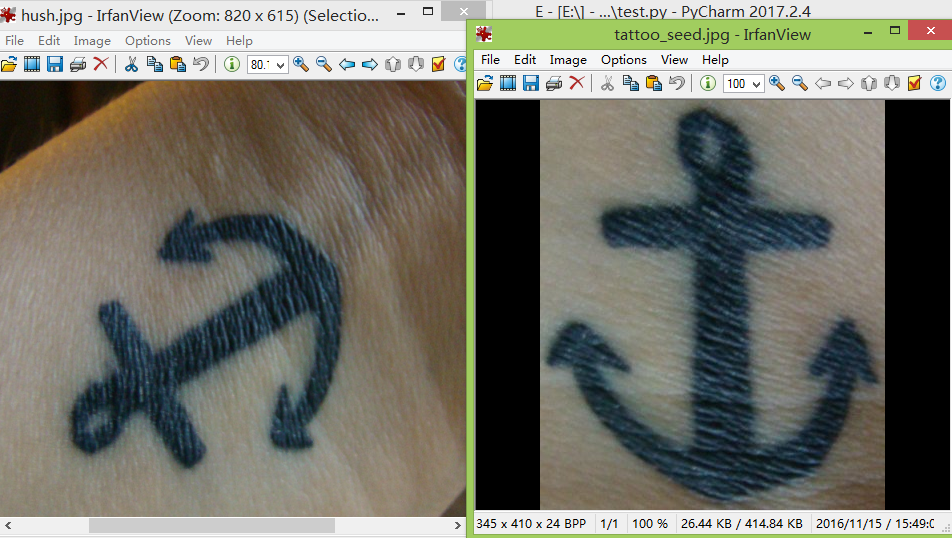展开全文Python 计算机视觉 人工智能
• 内容索引:VC/C++源码,图形处理,模板匹配法,手写数字识别　书中的一个经典实例，在此摘录出来，让C++此方面编程的朋友可以更容易的找得到，压缩包内有3种识别技术的算法实例，需要慢慢研究哦。
• 图像匹配 一些基本算法

万次阅读 多人点赞 2018-08-26 14:28:06

介绍

设S(x,y)是大小为mxn的搜索图像，T(x,y)是MxN的模板图像，分别如下图(a)、(b)所示，我们的目的是：在(a)中找到与(b)匹配的区域（黄框所示）。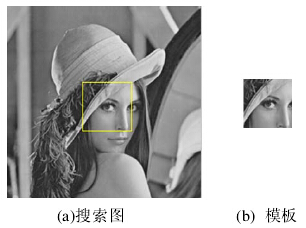算法思路

在搜索图S中，以(i,j)为左上角，取MxN大小的子图，计算其与模板的相似度；遍历整个搜索图，在所有能够取到的子图中，找到与模板图最相似的子图作为最终匹配结果。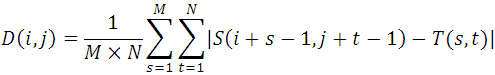其中：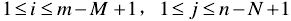算法评价：

优点：

①思路简单，容易理解（子图与模板图对应位置上，灰度值之差的绝对值总和，再求平均，实质：是计算的是子图与模板图的L1距离的平均值）。

②运算过程简单，匹配精度高。

缺点：

①运算量偏大。

②对噪声非常敏感。

———————————————————————————————————————————————————————————————————————————

介绍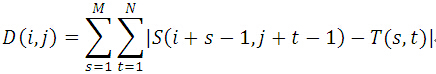算法实现

MATLAB代码

%%
clear all;
close all;
%%
[a b d]=size(src);
if d==3
src=rgb2gray(src);
end
if d==3
end
%%
N=n;%模板尺寸，默认模板为正方形
M=a;%代搜索图像尺寸，默认搜索图像为正方形
%%
dst=zeros(M-N,M-N);
for i=1:M-N         %子图选取，每次滑动一个像素
for j=1:M-N
temp=src(i:i+N-1,j:j+N-1);%当前子图
end
end
abs_min=min(min(dst));
[x,y]=find(dst==abs_min);
figure;
figure;
imshow(src);
hold on;
rectangle('position',[y,x,N-1,N-1],'edgecolor','r');
hold off;title('搜索图');

输出结果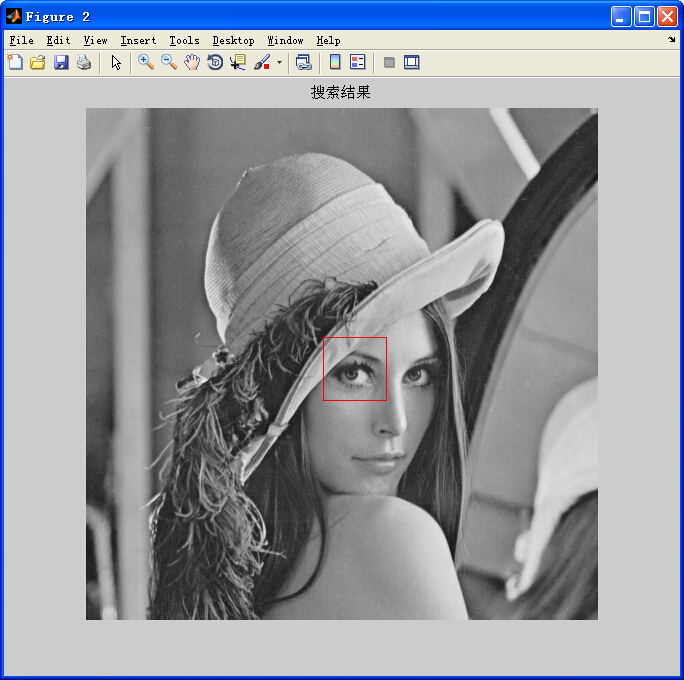——————————————————————————————————————————————————————————————————————————————

SSD算法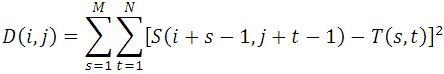——————————————————————————————————————————————————————————————————————————————

MSD算法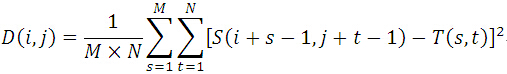————————————————————————————————————————————————————————————————————————————————

NCC算法

归一化积相关算法（Normalized Cross Correlation，简称NCC算法），与上面算法相似，依然是利用子图与模板图的灰度，通过归一化的相关性度量公式来计算二者之间的匹配程度。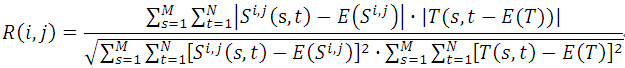其中，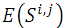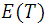分别表示(i,j)处子图、模板的平均灰度值。

————————————————————————————————————————————————————————————————————————

SSDA算法

序贯相似性检测算法（Sequential Similiarity Detection Algorithm，简称SSDA算法），它是由Barnea和Sliverman于1972年，在文章《A class of algorithms for fast digital image registration》中提出的一种匹配算法，是对传统模板匹配算法的改进，比MAD算法快几十到几百倍。

与上述算法假设相同：S(x,y)是mxn的搜索图，T(x,y)是MxN的模板图，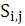是搜索图中的一个子图（左上角起始位置为(i,j)）。

显然：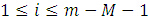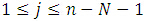SSDA算法描述如下：

①定义绝对误差：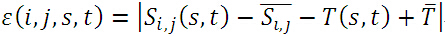其中，带有上划线的分别表示子图、模板的均值：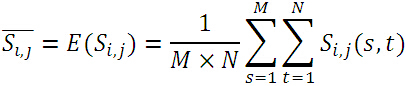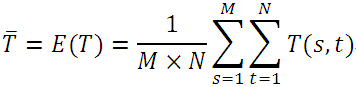实际上，绝对误差就是子图与模板图各自去掉其均值后，对应位置之差的绝对值。

②设定阈值Th；

③在模板图中随机选取不重复的像素点，计算与当前子图的绝对误差，将误差累加，当误差累加值超过了Th时，记下累加次数H，所有子图的累加次数H用一个表R(i,j)来表示。SSDA检测定义为：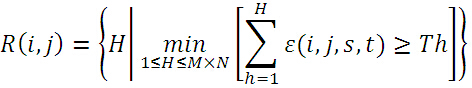下图给出了A、B、C三点的误差累计增长曲线，其中A、B两点偏离模板，误差增长得快；C点增长缓慢，说明很可能是匹配点（图中Tk相当于上述的Th，即阈值；I(i,j)相当于上述R(i,j)，即累加次数）。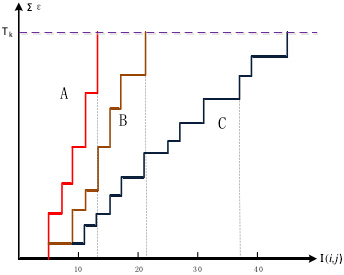④在计算过程中，随机点的累加误差和超过了阈值（记录累加次数H）后，则放弃当前子图转而对下一个子图进行计算。遍历完所有子图后，选取最大R值所对应的(i,j)子图作为匹配图像【若R存在多个最大值（一般不存在），则取累加误差最小的作为匹配图像】。

由于随机点累加值超过阈值Th后便结束当前子图的计算，所以不需要计算子图所有像素，大大提高了算法速度；为进一步提高速度，可以先进行粗配准，即：隔行、隔离的选取子图，用上述算法进行粗糙的定位，然后再对定位到的子图，用同样的方法求其8个邻域子图的最大R值作为最终配准图像。这样可以有效的减少子图个数，减少计算量，提高计算速度。

——————————————————————————————————————————————————————————————————————

SATD算法

MATLAB实现：

%//*****************************************

%//@filename:demo.m
%//@datetime:2015.08.20
%//@author:HJS
%//@e-mail:eleftheria@163.com
%//@blog:http://blog.csdn.net/hujingshuang
%//*****************************************
%%
clear all;
close all;
%%
M=size(src,1);%//搜索图大小
%%
hdm=zeros(M-N,M-N);%//保存SATD值
for i=1:M-N
for j=1:M-N
sw=(hdm_matrix*temp*hdm_matrix)/256;
hdm(i,j)=sum(sum(abs(sw)));
end
end
min_hdm=min(min(hdm));
[x y]=find(hdm==min_hdm);
title('模板');
figure;imshow(uint8(src));hold on;
rectangle('position',[y,x,N-1,N-1],'edgecolor','r');
title('搜索结果');hold off;
%//完

输出结果：以上便是几种常见的基于灰度的模板匹配算法。

展开全文图像匹配算法
• 特征提取与匹配在计算机视觉中是一个很重要的环节。比如人脸识别，目标跟踪，三维重建，等等都是先提取特征然后匹配特征最后执行后面的算法。因此学习特定提取和匹配是计算机视觉中的基础。本文将介绍FAST...

特征点提取与匹配在计算机视觉中是一个很重要的环节。比如人脸识别，目标跟踪，三维重建，等等都是先提取特征点然后匹配特征点最后执行后面的算法。因此学习特定点提取和匹配是计算机视觉中的基础。本文将介绍FAST特征点提取与匹配算法的原理，并使用Python不调用OpenCV包实现FAST特征点提取算法。

特征点提取到底是提取的是什么？

答：首先，提取的是角点，边缘。提取角点可以进行跟踪，提取边就可以知道图像发生了怎样的旋转。反正都是提取的是那些周围发生颜色明显变化的那些地方。这个也很容易想通，要是它周围全一样的颜色那肯定是物体的内部，一来没必要跟踪。二来它发生了移动计算机也无法判断，因为它周围都一样颜色计算机咋知道有没有变化。其次，提取的是周围信息（学术上叫做：描述子）。我们只要提到特征点提取就一定要想到提取完后我们是需要匹配的。为了判断这个点有没有移动，我们需要比较前后两帧图片中相同特征点之间是否有位移。为了判断是否是相同特征点那就要进行比对（匹配）。怎么比较两个特征点是否是同一个这就需要比较这两个特征点周围信息是否一样。周围信息是一样那就认为是同一个特征点。那么怎么比较周围信息呢？一般会把周围的像素通过一系列计算方式变成一个数字。然后比较这个数字是否相同来判断周围信息是否相同。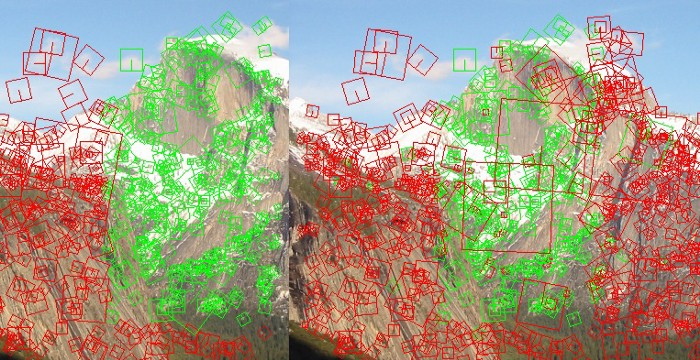所有特征提取与匹配算法通用过程

1. 找到那些周围有明显变化的像素点作为特征点。如下图所示，那些角点和边缘这些地方明显颜色变化的那些像素点被作为特征点。2. 提取这些特征点周围信息。一般是在当前这个点周围随机采样选几个像素点作为当前特征点的周围信息，或者画个圈圈进行采样。不同采样方法构成了不同算法。反正你想一个采样方法那你就创建了一种算法。

3. 特征点匹配。比如我要跟踪某个物体，我肯定是要先从这个物体提取一些特征点。然后看下一帧相同特征点的位置在哪，计算机就知道这个物体位置在哪了。怎么匹配？前面提到了我们第2步有提取当前特征点周围信息，只要周围信息一样那就是相同特征点。特征匹配也有很多种算法，最土的是前后两帧图片上的特征点一个一个的比对。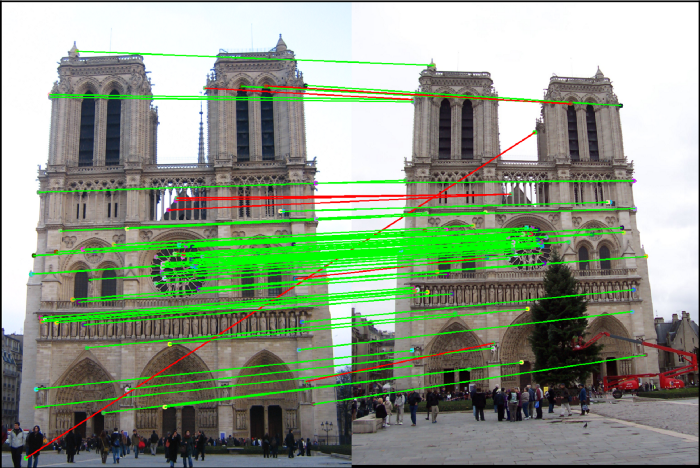记住学习任何特征提取与匹配算法都要时刻想起上面提到的三步骤。这样你不会太陷入那些书里面的细节中而学了很久都不懂。或者学完就忘。事实上那些算法非常简单，只不过你不知道他们各个步骤之间的联系是什么为什么这么设计。不知道这些当然就看不懂了。

FAST特征点提取算法

FAST (Features from Accelerated Segment Test)是一个特征点提取算法的缩写。这是一个点提取算法。它原理非常简单，遍历所有的像素点，判断当前像素点是不是特征点的唯一标准就是在以当前像素点为圆心以3像素为半径画个圆（圆上有16个点），统计这16个点的像素值与圆心像素值相差比较大的点的个数。超过9个差异度很大的点那就认为圆心那个像素点是一个特征点。那么什么叫做差异度很大呢？答：就是像素值相减取绝对值，然后我们设置一个数字只要前面那个绝对值大于这个数字，那就认为差异大。比如我设置阈值是3。第1个像素点的像素值是4，中间圆心像素值是10，然后10-4=6，这是大于阈值3的。所以第1个像素点算所一个差异度较大的像素点。就这样**统计1~16个中有多少个是和圆心相比差异度比较大的点。只要超过9个那就认为圆心是一个特征点。**是不是很简单？其实这些算法只要你知道他们想干嘛，你也可以设计一个不错的算法的。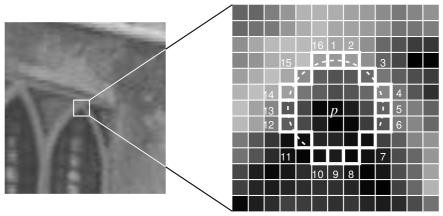为了简化问题我们构造一个带有角点的7x7的小图片，注意下面坐标轴单位是像素。（自己构造一些图片是检验我们实现的算法很重要的一个技能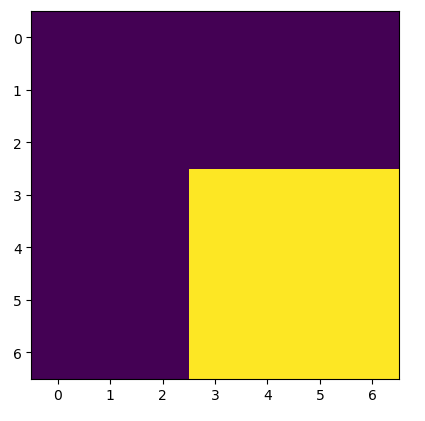然后使用bresenham算法画圆（如下图所示），可以看到周围有超过9个点与中心那个像素点的像素值很大差异。因此程序会判断当前圆心所在的像素点是关键点。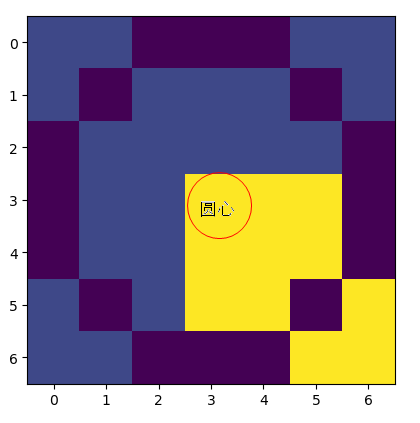现在稍微有一丝丝难度的是怎么画圆。因为这个圆它是一个像素一个像素的画。这个圆其实你自己可以随便设计一个算法画圆。今天我们要讲FAST算法当然还是介绍下他是怎么画圆的。他就用了最普通的图形学画圆算法（Bresenham 画圆法 ）。

其实到这里FAST算法我们就介绍完了。为了节省大家的时间（你的赞和关注是支持我分享的动力），我把Bresenham 画圆法也讲讲。

Bresenham 布雷森汉姆算法画圆的原理与编程实现教程

注意：Bresenham的圆算法只是中点画圆算法的优化版本。区别在于Bresenham的算法只使用整数算术，而中点画圆法仍需要浮点数。你不了解中点画圆法并没有任何影响，因为他们只是思想一样，但是思路并不是一样。

Bresenham也是根据待选的两个点哪个离圆弧近就下一步选哪个。它是怎么判断的呢？这两个点一定有一个在圆弧内一个在圆弧外。到底选哪个？Bresenham的方法就是直接计算两个点离圆弧之间的距离，然后判断哪个更近就选哪个。如下图所示：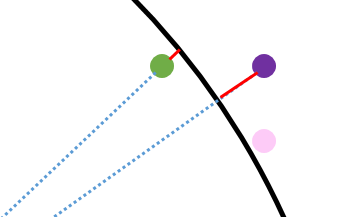那么怎么用数学量化他们离圆弧的距离呢？
答：前面我们提到了，当前粉红色这个点坐标为 ( x k , y k ) (x_k,y_k) ，下一步它有两种可能的走法绿色 ( x k − 1 , y k + 1 ) (x_k-1,y_k+1) ，紫色坐标为 ( x k , y k + 1 ) (x_k,y_k+1)
d 1 = ( x k − 1 ) 2 + ( y k + 1 ) 2 − r 2 d1 = (x_k-1)^2+(y_k+1)^2-r^2
d 2 = ( x k ) 2 + ( y k + 1 ) 2 − r 2 d2 = (x_k)^2+(y_k+1)^2-r^2
注意： d 1 = ( x k − 1 ) 2 + ( y k + 1 ) 2 − r 2 d1 = (x_k-1)^2+(y_k+1)^2-r^2 小于0的，因为绿色那个点一定在圆内侧。 d 2 = ( x k ) 2 + ( y k + 1 ) 2 − r 2 d2 = (x_k)^2+(y_k+1)^2-r^2 一定是大于等于0的，因为紫色那个点一定在圆外侧。

所以我们只用比较 P k = d 1 + d 2 P_k = d1+d2 到底是大于0还是小于0就能确定选哪个点了。大于0选绿色 ( x k − 1 , y k + 1 ) (x_k-1,y_k+1) 那个点（因为紫色那个点偏离圆弧程度更大）。小于0则选紫色 ( x k , y k + 1 ) (x_k,y_k+1) 那个点

好了Bresenham画圆法我讲完了

你或许会问，不对啊。我在网上看到的关于Bresenham画圆法的博客还有其他公式。确实我还有一个小细节没讲。你用上面的方法是已经可以画圆了，剩下的就是一些提高计算效率的小细节

P k = d 1 + d 2 = ( x k − 1 ) 2 + ( y k + 1 ) 2 − r 2 + ( x k ) 2 + ( y k + 1 ) 2 − r 2 P_k = d1+d2= (x_k-1)^2+(y_k+1)^2-r^2+(x_k)^2+(y_k+1)^2-r^2 这个公式走到下一步时候 P k + 1 = d 1 + d 2 P_{k+1} = d1+d2 又要重新计算。为了提高效率。人们就想能不能通过递推的方式来算 P k + 1 P_{k+1} ，即能不能找一个这样的公式 P k + 1 = P k + Z P_{k+1}=P_k+Z 提高计算效率。

这个也很简单，这个递推公式关键在于求Z。而我们变换下公式 P k + 1 = P k + Z P_{k+1}=P_k+Z 得到 Z = P k + 1 − P k Z=P_{k+1}-P_k
注意： P k = d 1 + d 2 = ( x k − 1 ) 2 + ( y k + 1 ) 2 − r 2 + ( x k ) 2 + ( y k + 1 ) 2 − r 2 P_k= d1+d2= (x_k-1)^2+(y_k+1)^2-r^2+(x_k)^2+(y_k+1)^2-r^2 我们已知的，而 P k + 1 P_{k+1} 这个根据 P k P_k 大于0还是小于0也可以算出来。

1. P k > = 0 P_k>=0 则证明靠近外侧的那个待选点 ( x k , y k + 1 ) (x_k,y_k+1) 离圆弧更远，所以我们下一步选的点是另外一个靠近内侧圆弧的那个点 ( x k − 1 , y k + 1 ) (x_k-1,y_k+1) 。也就是说第k+1步那个点 ( x k + 1 , y k + 1 ) = ( x k − 1 , y k + 1 ) (x_{k+1},y_{k+1})=(x_k-1,y_k+1)
Z = P k + 1 − P k = ( x k + 1 − 1 ) 2 + ( y k + 1 + 1 ) 2 − r 2 + ( x k + 1 ) 2 + ( y k + 1 + 1 ) 2 − r 2 − [ ( x k − 1 ) 2 + ( y k + 1 ) 2 − r 2 + ( x k ) 2 + ( y k + 1 ) 2 − r 2 ] = ( x k − 1 − 1 ) 2 + ( y k + 1 + 1 ) 2 − r 2 + ( x k − 1 ) 2 + ( y k + 1 + 1 ) 2 − r 2 − [ ( x k − 1 ) 2 + ( y k + 1 ) 2 − r 2 + ( x k ) 2 + ( y k + 1 ) 2 − r 2 ] = − 4 x k + 4 y k + 10 Z=P_{k+1}-P_k= (x_{k+1}-1)^2+(y_{k+1}+1)^2-r^2+(x_{k+1})^2+(y_{k+1}+1)^2-r^2 -[ (x_k-1)^2+(y_k+1)^2-r^2+(x_k)^2+(y_k+1)^2-r^2] \\ = (x_k-1-1)^2+(y_k+1+1)^2-r^2+(x_k-1)^2+(y_k+1+1)^2-r^2 -[ (x_k-1)^2+(y_k+1)^2-r^2+(x_k)^2+(y_k+1)^2-r^2]\\ =-4x_k+4y_k+10 。所以 P k + 1 = P k − 4 x k + 4 y k + 10 P_{k+1}=P_k-4x_k+4y_k+10
2. P k < 0 P_k<0 时，们下一步选的点是另外一个靠近内侧圆弧的那个点是 ( x k , y k + 1 ) (x_k,y_k+1) 。也就是说第k+1步那个点 ( x k + 1 , y k + 1 ) = ( x k , y k + 1 ) (x_{k+1},y_{k+1})=(x_k,y_k+1) 。我们看看现在的Z是多少。
Z = P k + 1 − P k = ( x k + 1 − 1 ) 2 + ( y k + 1 + 1 ) 2 − r 2 + ( x k + 1 ) 2 + ( y k + 1 + 1 ) 2 − r 2 − [ ( x k − 1 ) 2 + ( y k + 1 ) 2 − r 2 + ( x k ) 2 + ( y k + 1 ) 2 − r 2 ] = ( x k − 1 ) 2 + ( y k + 1 + 1 ) 2 − r 2 + ( x k ) 2 + ( y k + 1 + 1 ) 2 − r 2 − [ ( x k − 1 ) 2 + ( y k + 1 ) 2 − r 2 + ( x k ) 2 + ( y k + 1 ) 2 − r 2 ] = 4 y k + 6 Z=P_{k+1}-P_k= (x_{k+1}-1)^2+(y_{k+1}+1)^2-r^2+(x_{k+1})^2+(y_{k+1}+1)^2-r^2 -[ (x_k-1)^2+(y_k+1)^2-r^2+(x_k)^2+(y_k+1)^2-r^2] \\ = (x_k-1)^2+(y_k+1+1)^2-r^2+(x_k)^2+(y_k+1+1)^2-r^2 -[ (x_k-1)^2+(y_k+1)^2-r^2+(x_k)^2+(y_k+1)^2-r^2]\\ =4y_k+6 。所以 P k + 1 = P k + 4 y k + 6 P_{k+1}=P_k+4y_k+6
注意：根据初始点为(r,0)来计算 P k P_k 的初始值= − 2 r + 3 -2r+3
# -*- coding: utf-8 -*-
"""
Created on Sun Jul 21 15:02:36 2019
Bresenham画圆法实现
博客教程地址：
https://blog.csdn.net/varyshare/article/details/96724103
@author: 知乎@Ai酱
"""
import numpy as np
import matplotlib.pyplot as plt

img = np.zeros((105,105)) # 创建一个105x105的画布

def draw(x,y):
"""
绘制点(x,y)
注意：需要把(x,y)变换到数组坐标系（图形学坐标系）
因为数组(0,0)是左上，而原先坐标系(0,0)是中心点
而且数组行数向下是增加的。
"""
# 平移原点
x += int(img.shape/2)
y += int(img.shape/2)
#
img[-y,x] = 1
pass

r_pixel = 50 # 圆的半径,单位：像素
# 初始化,画第一个点，从水平最右边那个点开始画
(x,y) = (r_pixel,0)

"""
从定义来讲就是
P_k=d1+d2
d1 = 第1个下一步待选点离圆弧的距离（负数）
d2 = 第2个下一步待选点离圆弧的距离（正数）
但是为了提高效率通常使用递推来求P_{k+1}=P_k + 一个数
"""
P_k = -2*r_pixel + 3

# 迭代的求完1/8圆弧
while x>=y:
# 下一步有两个待选点，具体选哪个要看P_k>0 或 <0
if P_k>=0:# 外侧候选点偏离圆弧更远
P_k_next =  P_k - 4*x + 4*y + 10
(x_next,y_next) = (x-1, y+1)
else:# 内侧候选点偏离圆弧更远
P_k_next =  P_k + 4*y + 6
(x_next,y_next) = (x, y+1)
# 对称法画其他地方
draw(x,y)
draw(-x,y)
draw(x,-y)
draw(-x,-y)

draw(y,x)
draw(y,-x)
draw(-y,x)
draw(-y,-x)
# 更新坐标和P_k
(x,y) = (int(x_next),int(y_next))
P_k = P_k_next
pass
# 绘制图片
plt.imshow(img)

程序运行的结果为：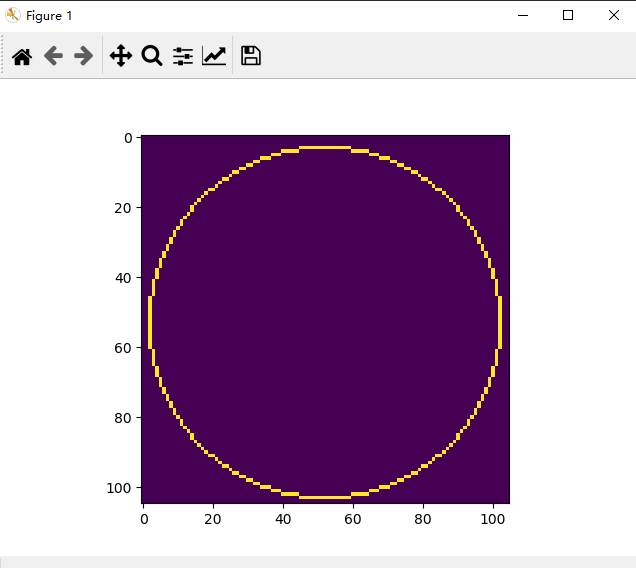上图的半径是40像素，当然FAST画圆的半径是3像素.我们只用修改一行设置半径的代码为r_pixel = 3 # 圆的半径,单位：像素。半径为3像素的圆如下图所示：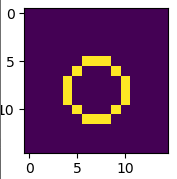使用OpenCV库中的FAST特征点检测算法

'''
Features from Accelerated Segment Test (FAST)
Python实现，
从0开始，最原始最简单的FAST特征点提取方法（无金字塔采样）
代码地址：https://github.com/varyshare/easy_slam_tutorial/tree/master/feature_extract
欢迎fork参与这个开源项目,star这项目
教程地址：https://blog.csdn.net/varyshare/article/details/96430924
代码没有怎么经过优化，所以会有0.8s左右的卡顿
'''
import numpy as np
import matplotlib.pyplot as plt
import cv2 # 用于读取图片

# 1. 读取图片(为了简化问题我就直接构造一个数组作为图片)

# 2. 设置参数
# 设置灰度值相差多大才算较大差异，
# 以及周围点超过多少个高差异点才算当前中心像素点是关键点
h_gray_scale = 20 # 在ORB特征提取中使用的FAST像素差阈值默认是20
k_diff_point = 9 # 超过k_diff_point个差异那就认为是关键点（周围共16个点）
r_pixel = 3 # 获取周围像素所用的圆的半径,单位：像素

# 3. 遍历所有的像素进行检测关键点
def bresenham_circle():
"""
return: 圆周上所有的点相对圆心的坐标列表。
即，返回圆心在(0,0)处时圆周上各点的坐标。
返回一个r_pixel*r_pixel的矩阵，圆周上的点标记为1，其他地方标记为0
"""

def save(x,y):
"""
把(x,y)加入到结果列表中
注意：需要把(x,y)变换到数组坐标系（图形学坐标系）
"""
pass

# 初始化,画第一个点，从水平最右边那个点开始画
(x,y) = (r_pixel,0)

"""
从定义来讲就是
P_k=d1+d2
d1 = 第1个下一步待选点离圆弧的距离（负数）
d2 = 第2个下一步待选点离圆弧的距离（正数）
但是为了提高效率通常使用递推来求P_{k+1}=P_k + 一个数
"""
P_k = -2*r_pixel + 3

# 迭代的求完1/8圆弧
while x>=y:
# 下一步有两个待选点，具体选哪个要看P_k>0 或 <0
if P_k>=0:# 外侧候选点偏离圆弧更远
P_k_next =  P_k - 4*x + 4*y + 10
(x_next,y_next) = (x-1, y+1)
else:# 内侧候选点偏离圆弧更远
P_k_next =  P_k + 4*y + 6
(x_next,y_next) = (x, y+1)
# 对称法画这对称的8个地方
save(x,y)
save(-x,y)
save(x,-y)
save(-x,-y)

save(y,x)
save(y,-x)
save(-y,x)
save(-y,-x)
# 更新当前坐标和P_k
(x,y) = (int(x_next),int(y_next))
P_k = P_k_next
pass

# 先bresenham算法算出半径为r_pixel时圆周上的点相对圆心的坐标
def key_point_test(_row,_col):
"""
检测像素点(_row,_col)是否是关键点。
满足关键点只有一个条件：周围16个像素点与中心像素点相比
差异度较大(>h_gray_scale)的像素点个数超过k_diff_point个
return: boolean
"""
# 获取以(_row,_col)为几何中心的7x7正方形区域内的像素值
surround_points = img[_row-3:_row+3+1,_col-3:_col+3+1]

# 获取圆周上的点与圆心的像素差值的绝对值
_dist = np.abs((surround_points - img[_row,_col])) * masked_canvas

if (_dist>h_gray_scale).sum()> k_diff_point:
return True
else:
return False

key_point_list = []

for row in range(r_pixel,img.shape-r_pixel):
for col in range(r_pixel,img.shape-r_pixel):

if key_point_test(row,col):
key_point_list.append(cv2.KeyPoint(x=row,y=col,_size=1))
else:
continue

pass
pass

img_with_keypoints = cv2.drawKeypoints(img,key_point_list,outImage=np.array([]),color=(0,0,255))
cv2.imshow("show key points",img_with_keypoints)
cv2.waitKey(0)

下图是我们对一个小图片进行检测程序运行的结果，可以看到最明显的几个角点找到了，但是也有几个点漏掉了。这是因为我们设置16个点中超过9个点和中心点不同这个数值9对于这张图来说大了些。你如果设置为7那就都能检测到了。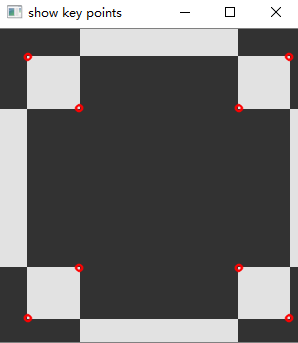参考文献：
 https://medium.com/software-incubator/introduction-to-feature-detection-and-matching-65e27179885d
 https://docs.opencv.org/3.0-beta/doc/py_tutorials/py_feature2d/py_fast/py_fast.html#fast
 https://medium.com/software-incubator/introduction-to-fast-features-from-accelerated-segment-test-4ed33dde6d65

展开全文• 这都牵扯到一种技术，那就是数据关联，而匈牙利算法就是解决此类问题典型的算法，也是今天本文的主题。 我们感性的认为目标之间的匹配好像一目了然的样子，但是计算机可不这样认为。计算机是理性的，如果要处理...

在软件开发领域，任务指派和数据关联是一种常见业务需求，比如买卖订单的匹配，共享出行的人车匹配，及自动驾驶领域中目标追踪。

这都牵扯到一种技术，那就是数据关联，而匈牙利算法就是解决此类问题最典型的算法，也是今天本文的主题。

我们感性的认为目标之间的匹配好像一目了然的样子，但是计算机可不这样认为。计算机是理性的，如果要处理问题，一般我们会用数据结构来表示数据，栈、队列、树、图都很常用。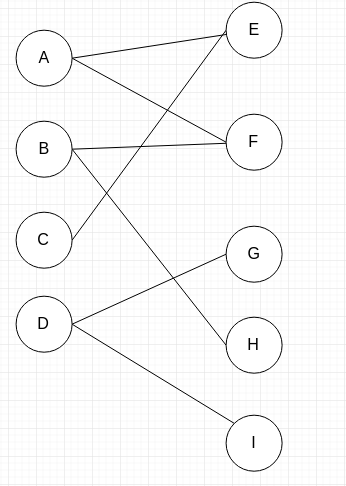上面的形式，其实也可以用 表示，所以它等同于下面的形式。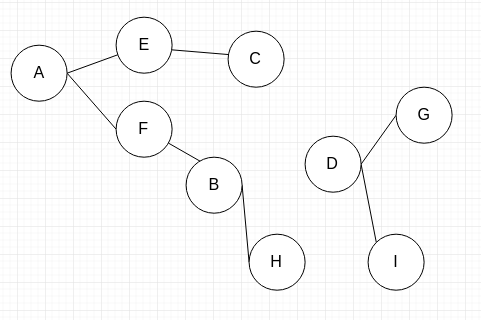不过，这是一种特殊的图，叫做二分图

二分图图最大的特点就是，图中的顶点可以分别划分到两个 Set 当中，每个 Set 中的顶点，相互之间没有边连接。我们假设一个集合 X,它的元素可以有 {A,B,C,D}。
假设一个集合 Y,它的元素可以有{E,F,G,H,I}。

恰好这个图中的所有顶点都可以分配到这两个集合当中，每个集合中的顶点互相没有连接。

这样我们不难理解，匹配问题就可以转换成图的形式，用图论中的算法来解决问题，匈牙利算法就是求这样的二分图的匹配。

匹配

匹配是专业名词，它是指一组没有公共端点的边的集合。

按照定义，匹配里面的元素是边。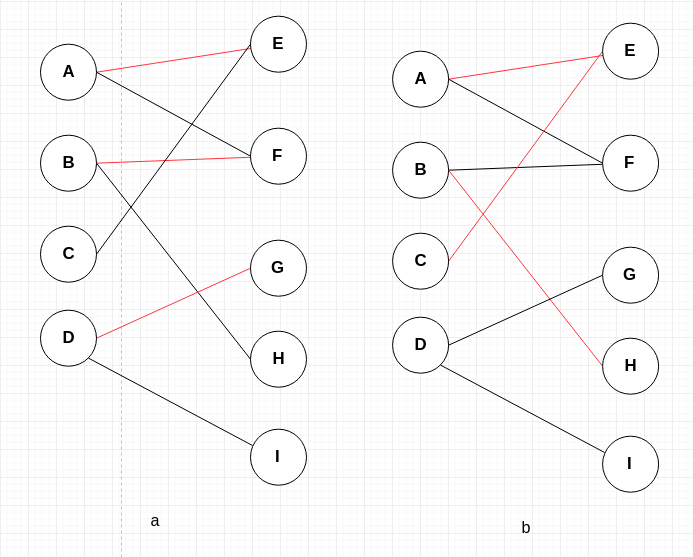上图 a 中的红线可以是一个集合，而 b 不是。

最大匹配

按照定义，我们很容易知道，一个图中可以有许多匹配，而包含边数最多的那个匹配，我们称之为最大匹配。

完美匹配

如果一个匹配，它包含了图中所有的顶点，那么它就是完美匹配。

完备匹配

完备匹配的条件没有完美匹配那么严苛，它要求一个匹配中包含二分图中某个集合的全部顶点。比如，X 集合中的顶点都有匹配边。

匈牙利算法就是要找出一个图的最大匹配。

算法思想

其实匈牙利算法如果要感性理解起来是很容易的，核心就在于

冲突和协调

我们看看下图：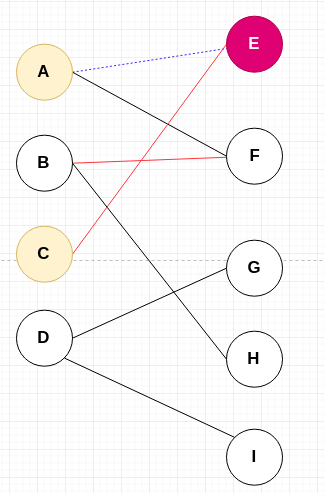黑线表示可以匹配，
红线表示当前匹配，
蓝色的虚线表示取消匹配

那么匈牙利算法是怎么一个过程呢？

第 1 步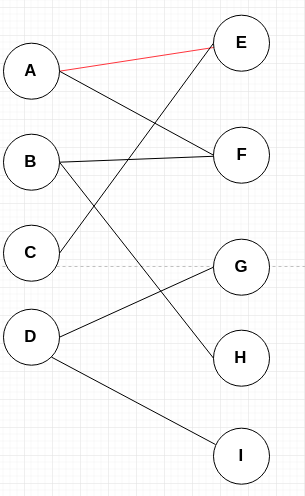从顶点 A 开始，E 点可以匹配，那么就直接匹配。并将边 AE 加入到匹配中。
第 2 步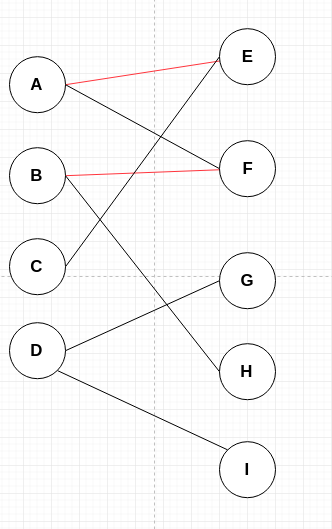从 B 点开始，F 点可以匹配，所以将 BF 边也加入到匹配中。
第 3 步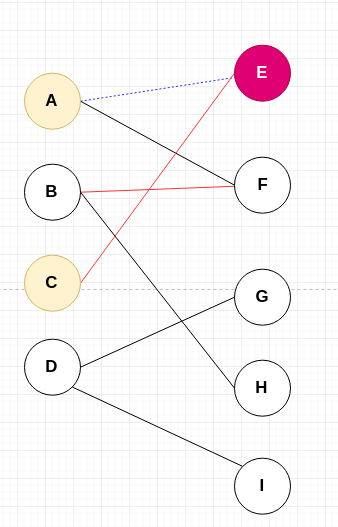当 C 点开始寻求匹配时，可以发现 CE 和 AE 起冲突了。
前面讲到了冲突和协调两个关键词。
匈牙利算法是让CE 先匹配， AE 取消匹配，然后让 A 尝试重新匹配。

第 4 步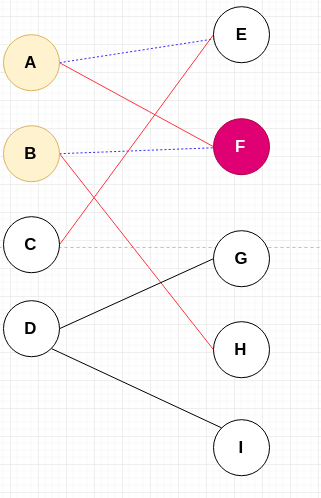A 在再次寻找匹配的道路上，发现 AF 和 BF 又冲突了，所以需要递归协调下去。
这时，让 AF 匹配，BF 断开连接，让 B 点去重新寻找匹配。
第 5 步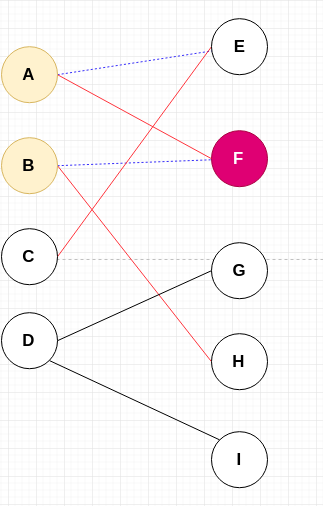B 点寻求匹配的过程，没有再遇到冲突，所以，BH 直接匹配。

需要注意的是，这条路径是从顶点 C 出发的，发生了 2 次冲突，协调了两次，最终协调成功。

如果协调不成功的话，CE 这条路就不成功，它需要走另外的边，但是上图中 C 只和 E 有可能匹配，所以，如果协调不成功，C 将找不到匹配。

第 6 步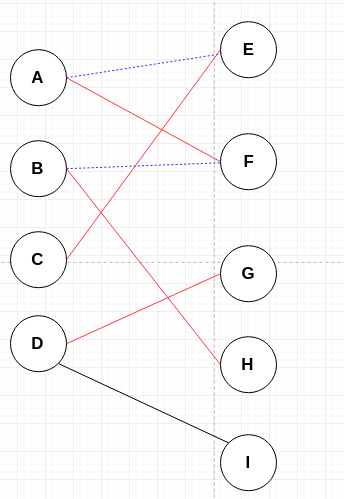从 D 点开始寻求匹配时，直接可以和 DG 匹配。

最终匈牙利算法就结束了，找到了最大匹配。
最终的结果就是：

A <--> F
B <--> H
C <--> E
D <--> G

相信到这里后，大家都弄懂了算法的原理，但是这并不代表你能写好代码。

弄懂了道理，不代表你能写好代码，不信，你先不看下面的内容自己试一试怎么写出来。

匈牙利算法的思想就是：
1.如果没有冲突，按照正常的连接
2.有冲突的话，就冲突的顶点协调，递归下去。

所以，递归很重要，我们就可以写出如下的代码，本文示例用 Python 编写，其它编程语言其实相差也不大。

# G 是临接表的方式表达图形
G={}
G = {0,1}
G = {1,3}
G = {0}
G = {2,4}

match_list = [-1,-1,-1,-1,-1]

label_x = ['A','B','C','D']
label_y = ['E','F','G','H','I']

# v 代表当前的 x 集合中的顶点
# current 代表 y 集合中起冲突的顶点，如果为 -1 则代表没有冲突
def match(v,current):
for i in G[v]:
if i == current:continue

# 如果可以直接匹配，或者是协调一下就可以匹配，那么就匹配成功，并做标记
if match_list[i] == -1 or match(match_list[i],i):
match_list[i] = v
return True

return False

def hungarian():

# 访问 X 集合的顶点
for i in range(G.__len__()):
# 对集合中的顶点逐个匹配
match(i,-1)

for i in range(match_list.__len__()):
if match_list[i] == -1:continue
print("%s <--match--> %s:" %(label_x[match_list[i]],label_y[i]))

if __name__ == "__main__":

hungarian()

代码很简单，详情见注释，打印结果如下：

C <--match--> E:
A <--match--> F:
D <--match--> G:
B <--match--> H:

到这里，就可以了，匈牙利算法的思想和代码编写我们都掌握了。

但是，文章最后，我还是想用更学术的方式去描述和编写这个算法。

用学术的目的是让我们显得更科班，不能总是野路子凭灵感去弄是吧？

学术化的目的是为了让我们的思维更严谨，夯实我们的技术基础，这样遇到新的问题时，我们能够泛化解决它。

交替路与增广路

我们现在站在结果处回溯。

上面求得匈牙利的匹配结果如下图：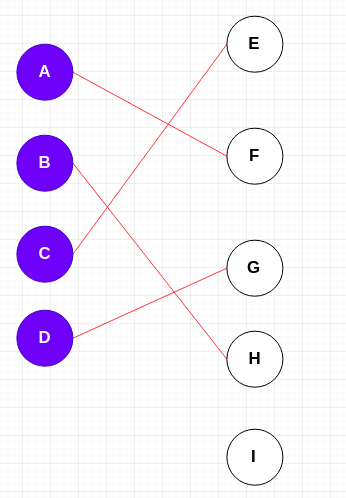我们可以稍作变化。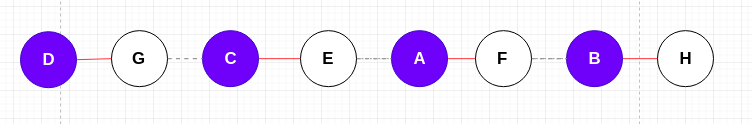我们可以观察到，匈牙利算法要求得的匹配结果，可以用上图形式表示。

红实线表示两顶点匹配，灰色的虚线表示未匹配。

上面的路径中匹配的边和未匹配的边交替出现。

所以，我们的目标是去构建这样一条路径。

这涉及到两个概念：交替路和增广路。

什么是交替路？

从未匹配的顶点出发，依次经过未匹配的边，匹配的边，未匹配的边，这样的路径就称为交替路。

重点是未匹配的点和未匹配的边开始

什么是增广路？

从未匹配的顶点出发，按照交替路的标准寻找顶点，如果途经了另外一个未匹配的点，那么这条路径就是增广路。

增广路有一些重要的特征：

1. 增广路中为匹配的边的数量要比匹配的边的数量多 1 条。
2. 增广路取反(匹配的边变成未匹配，未匹配的边变成匹配)后，匹配的边的数量会加 1，从而达到匹配增广的目的。

而匈牙利算法其实就是就可以看做是一个不断寻找增广路的过程，直到找不到更多的增广路

下面图例说明：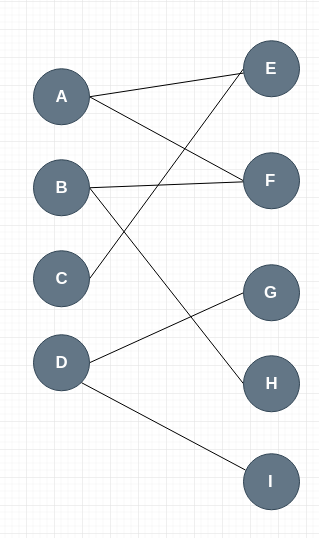上面是二分图。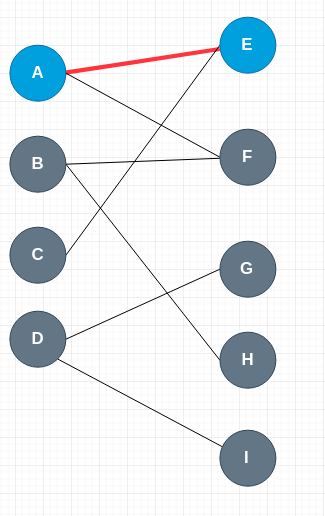首先，从顶点 A 开始，如果要寻求增广路，它应该先走未匹配的边，可以选择 AE,因为 E 点也未匹配，所以 A --> E 符合增广路的定义，它就是一条增广路。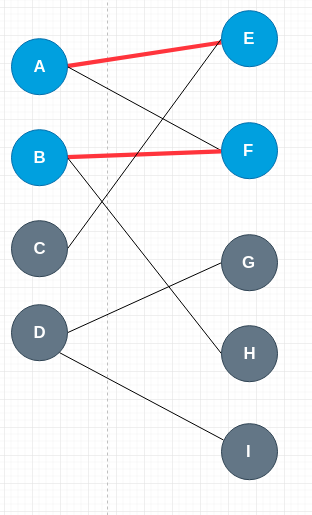再从 B 点找增广路，因为路径 BF 没有匹配，所以 B --> F 也是一条增广路。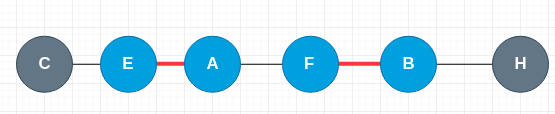再从 C 点寻找增广路，它走未匹配的边，CE,然后走 EA，再走 AF,再走 FB,最后 BH,因为 H 是未匹配的点，所以 C–>E–>A–>F–>B–>H 就是一条增广路，如上图。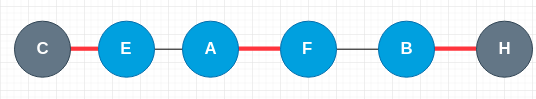我们知道对增广路取反，匹配的边数量会加 1，那么我们果断这样做。

也就是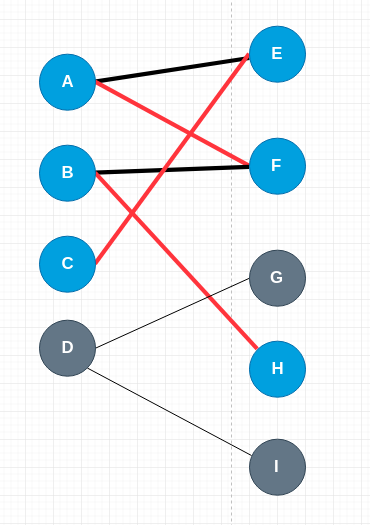我们再对 D 顶点寻找增广路。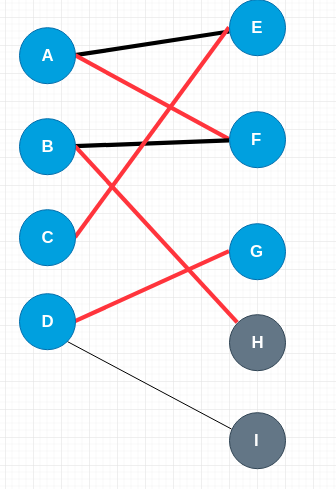很容易就找到了，自此匈牙利算法就此结束，途中的匹配就是最大匹配。

我们看到，以增广路的方式描述算法其实就包括了文章前面提到的冲突与协调这种感性的思想，因为它显得更加科学和条理清楚。

下面是以增广路的方式实现的代码。

import numpy as np

# G 是临接表的方式表达图形
G={}
G = {0,1}
G = {1,3}
G = {0}
G = {2,4}

match_list = [-1,-1,-1,-1,-1]

label_x = ['A','B','C','D']
label_y = ['E','F','G','H','I']

y_in_path = [False,False,False,False,False]

def find_agument_path(v):

for i in G[v]:
# 已经在交替路中出现的点就不需要匹配，避免死循环，例如 C 点需求增广路过程中，A 点不应该和E点再匹配
if not y_in_path[i]:
y_in_path[i] = True
if (match_list[i] == -1 or find_agument_path(match_list[i])):
match_list[i] = v
return True
return False

def hungarian():

for i in range(G.__len__()):
global y_in_path
# 清空交替路
y_in_path = [False,False,False,False,False]
find_agument_path(i)

for i in range(match_list.__len__()):
if match_list[i] == -1:continue
print("%s <--match--> %s:" %(label_x[match_list[i]],label_y[i]))

if __name__ == "__main__":

hungarian()

打印结果如下：

C <--match--> E:
A <--match--> F:
D <--match--> G:
B <--match--> H:

也许有同学会问，怎么没有看见对增广路取反？

其实，match_list 数组就保存了匹配关系，当改变其中的值顶点的匹配关系就变了，并不需要用一个真正的链表来表示增广路。

展开全文匈牙利算法 最大匹配 算法
• halcon 形状匹配

万次阅读 多人点赞 2018-10-31 14:01:57
如果检测对象几乎是对称的，那么就应该限制角度范围，否则模板会在不同的角度上找到多个最匹配的模板，十字形状或者正方形形状必须将角度范围限定在90°，对于长方形，应该限制在180°，圆的话应该是0°。...halcon
• Distinctive Image Features from Scale...通过使用快速最近邻居算法将各个特征与来自已知对象的特征数据库匹配，然后进行霍夫变换以识别属于单个对象的聚类，最后通过最小二乘解决方案对一致的姿势参数进行验证。 ...SIFT
• 模板匹配

万次阅读 2016-06-23 16:39:17
在模式识别的各种方法中，模板匹配最容易的一种，其数学模型易于建立，通过模板匹配对数字图像模式识别有助于我们了解数学模型在数字图像中的应用。 二、模板匹配算法 1、相似性测度求匹配  模板匹配的实际操作...
• 找到所有特征后，要去除低对比度和不稳定的边缘效应的，留下具有代表性的关键（比如，正方形旋转后变为菱形，如果用边缘识别，4 条边就完全不一样，就会错误；如果用角识别，则稳定一些）。去除这些的...图形图像处理
• 立体匹配中的全局匹配（一）动态规划笔记

万次阅读 多人点赞 2016-09-19 16:17:38
近来研究立体匹配，从入门开始，先学习一些基本的算法思想。 立体匹配算法中，全局匹配是一个很重要的部分，利用图像的全局约束信息，对局部图像的模糊不敏感，它的计算代价很高。全局匹配算法通过构建全局能量函数...动态规划 算法
• **Outline:** 1、Global Image Features (Hough Transform)霍夫变换 2、角检测 3、SIFT特征 4、Learning with many simple features计算机视觉 图像处理
• Qt 之图形视图框架

万次阅读 多人点赞 2016-07-20 16:59:13
简述图形视图（Graphics View）提供了一个用于管理和交互大量自定义的二维图形对象（Item），以及一个支持缩放和旋转操作的视图部件用于显示这些视图项。框架包括一个事件传播架构，支持scene中的items进行精确的双...Qt QGraphics Qt图形视图 Qt图形视图框架 Qt视图
• 如何快速成长为图形学工程师

千次阅读 多人点赞 2018-01-19 00:00:00
本文来自作者 姜雪伟 在 GitChat 上分享 「如何快速成长为图形学工程师」，「阅读原文」查看交流实录。「文末高能」编辑 | 哈比目前 IT 市场出现了各路诸侯争霸局面，从大的方向说分为三类：PC 端、移动端、VR/...
• 数学在计算机图形学中的应用 刘利刚 中国科技大学 “学习计算机图形学需要多少的数学？”这是初学者经常问的问题。...狭义的计算机图形学指的是传统的三维建模，绘制，动画等...相对来讲，计算机图形学能看到学...
• 图像相似性匹配 快速算法

万次阅读 2018-10-18 15:59:06
方法1： 关键点匹配（Keypoint Matching） 一张图像的某些部位可能蕴含比其它部位更多的信息，如边缘，角。因此我们可以利用一些算法提取图像的关键信息进行比较。SIFT，ORB，SURF，GIST都是此类提取关键信息...
• 数据来源来源：网络资源，其实也比较容易找，比如人大经济论坛。但为了避免相关的版权争议，我重新写了一个do文件，处理的方法和变量也有改变。举例：接受培训对于工资的影响分析思路：(1)验证选择性的存在；(2)倾向...
• 立体匹配导论

万次阅读 2016-05-29 12:26:08
如果在两幅图像中，匹配点的顺序不同，那么在场景中的匹配点就是遮挡。（整体有最低的误判率和最低的触发率） 遮挡约束（OCC） 假设视差图中的不连续区为遮挡区，所以，要找到遮挡区域，只需要找到视差图中的不...计算机视觉
• 基于形状的模板匹配

万次阅读 2015-03-25 21:06:27
前段时间一直在图像模板匹配。需要对旋转模板进行匹配，并且对速度精度都有较高的要求。OpenCV里面并没有较好的解决方法。 cvMatchTemplate( const CvArr* image, constCvArr* templ,CvArr* result,int method ) ...模板匹配 halcon
• 目录halcon手绘形状匹配模板手绘形状匹配模板主要算子解析draw_...最近了个项目，遇到一个问题，就是在创建形状匹配模板时候，干扰太多，像麻绳一样。 使用自定义区域去消除吧，因为轮廓长，边缘干扰距离近，操作halcon 图像处理 工业视觉
• 基于最小生成树的实时立体匹配算法简介

千次阅读 热门讨论 2016-05-29 13:30:11
图割，置信传播等全局优化立体匹配算法，由于运算过程中需要迭代求精，运算时间长，无法达到实时计算立体匹配的需求，然而实时性需求却广泛存在立体匹配的应用场景中。很多基于局部匹配的算法虽然运算时间短，但由于...计算机视觉
• Altium Designer -- 差分布线和阻抗匹配

万次阅读 多人点赞 2016-03-16 13:28:01
一、PCB 差分布线操作参看：Altium Designer -- 精心总结PCB 差分布线已经讲的很清楚了，在此不介绍。二、差分布线优缺点参看：实际运用中差分信号线的分析和 LAYOUT 参看：差分信号 -- 维基百科（1） 差分信号...
• 笔者介绍：姜雪伟，IT公司技术合伙人，IT高级讲师，CSDN社区专家，特邀编辑，畅销书作者，...市面上，跨平台引擎使用的底层图形库都是用OpenGL，很多人都认为OpenGL是一个API(Applicatoin Programming Interface,应用程cocos2d-x opengl 图形
• 射频基础之阻抗匹配与Smith图

万次阅读 多人点赞 2017-09-06 10:57:09
对电子设备互连来说，例如信号源连放大器，前级连后级，只要后一级的输入阻抗大于前一级的输出阻抗5-10倍以上，就可认为阻抗匹配良好；对于放大器连接音箱来说，电子管机应选用与其输出端标称阻抗相等或接近的音箱，...阻抗匹配 smith圆
• 本文介绍了连续图像和数字图像直方图匹配（直方图规定化）的原理、处理过程，并提供了案例进行了讲解。可以看到，直方图均衡处理是直方图匹配的一个重要桥梁。 最后，对于直方图规定化这个翻译个人觉得很low，个人...python 人工智能 数字图像处理 直方图匹配 直方图规定化
• 轮廓匹配、识别

千次阅读 2020-10-21 16:10:33
OpenCv轮廓高级应用（轮廓匹配，几何直方图） 最近再次用到了opencv轮廓，在这里结合作者冰山一角的博客（http://www.cnblogs.com/slysky/）以及自己的体会在此稍加说明。其程序主要参见冰山一角的Blog，遗憾的是...
• 对于复杂一点识别的可能还涉及到大量的识别训练，最后的匹配比较分类等等。后续可能会介绍文字识别、人脸识别等等。 图形识别 图形识别指的是对常见的几何图形进行识别，它通过opencv进图形处理（二值化，图片灰度...
• 利用python破解图形验证码

千次阅读 2019-04-23 16:13:03
提供分级结果，你可以查看接近的多个匹配 对于无法识别的东西只要加入到搜索引擎中，马上就能识别了。 当然它也有缺点，例如分类的速度比神经网络慢很多，它不能找到自己的方法解决问题等等。 关于向量空间...python 验证码 pillow PIL 图形验证码
• 一小时学懂阻抗匹配

万次阅读 多人点赞 2018-04-16 15:47:35
串联匹配常用的终端匹配方法。它的优点是 功耗小 ，不会给驱动器带来额外的直流负载，也不会在信号和地之间引入额外的阻抗，而且只需要一个电阻元件。 常见应用： 一般的CMOS、TTL电路的阻抗匹配。USB信号也...
• 图像采样： 自己写了一个程序，从所要识别网站上爬取验证码图片，采集的图片越多越好，训练的样本多了训练出的模型才能有很强的泛性，如果样本数太少模型容易出现过拟合现象，训练出的模型准确度就会很低。...
• GUI图形界面编程基础知识

千次阅读 2021-11-09 23:23:34
文章目录一、GDI概述二、OpenGL三、什么是DirectX?...Qt简介Qt 可以什么？七、游戏开发引擎1、Unity 3D2、虚幻引擎 一、GDI概述 GDI在全称是Graphics Device Interface，即图形设备接口。是图形显示与实gui opengl directx mfc...